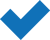# AI – Linear & Logistic Regression with Excel, R and Python 2017

£359.00

Our ’Linear & Logistic Regression’ e-Learning course will teach you how to build robust linear models and do logistic regressions in Excel, R, and Python that will be automatically applicable in real world situations. Supplemental Materials included!

You’ll learn about topics such as: understanding random variables, cause-effect relationships, maximum likelihood estimation, and so much more. Follow along with the experts as they break down these concepts in easy-to-understand lessons.

SKU: EC4Y0251 Category: Tag:

Our ’Linear & Logistic Regression’ e-Learning course will teach you how to build robust linear models and do logistic regressions in Excel, R, and Python that will be automatically applicable in real world situations. Supplemental Materials included!

You’ll learn about topics such as: understanding random variables, cause-effect relationships, maximum likelihood estimation, and so much more. Follow along with the experts as they break down these concepts in easy-to-understand lessons.

What is Linear & Logistic Regression?

In Statistics, Linear regression refers to a model that can show relationship between two variables. Logistic regression is another generalised linear model (GLM) procedure using the same basic formula however features differences to produce a more limited amount of outcomes.

#### What’s Included?Unlimited access for 12 monthsAccess anywhere, any timeFast effective training, written and designed by industry expertsTrack your progress with our Learning Management SystemUnlimited supportSave money, time and travel costsLearn at your own pace and leisureEasier to retain knowledge and revise topics than traditional methodsExam preparation quizzes, tests and mock exams to ensure that you are 100% ready

£359.00

## Modules

Course Length: 5 Hours

Chapter 01: Introduction

You, This Course, & Us!

Chapter 02: Connect the Dots with Linear Regression

Using Linear Regression to Connect the Dots
Two Common Applications of Regression
Extending Linear Regression to Fit Non-linear Relationships

Chapter 03: Basic Statistics Used for Regression

Understanding Mean & Variance
Understanding Random Variables
The Normal Distribution

Chapter 04: Simple Regression

Setting up a Regression Problem
Using Simple Regression to Explain Cause-Effect Relationships
Using Simple Regression for Explaining Variance
Using Simple Regression for Prediction
Interpreting the results of a Regression
Mitigating Risks in Simple Regression

Chapter 05: Applying Simple Regression

Applying Simple Regression in Excel
Applying Simple Regression in R
Applying Simple Regression in Python

Chapter 06: Multiple Regression

Introducing Multiple Regression
Some Risks inherent to Multiple Regression
Benefits of Multiple Regression
Introducing Categorical Variables
Interpreting Regression results – Adjusted R-squared
Interpreting Regression results – Standard Errors of Coefficients
Interpreting Regression results – t-statistics & p-values
Interpreting Regression results – F-Statistic

Chapter 07: Applying Multiple Regression using Excel

Implementing Multiple Regression in Excel
Implementing Multiple Regression in R
Implementing Multiple Regression in Python

Chapter 08: Logistic Regression for Categorical Dependent Variables

Understanding the need for Logistic Regression
Setting up a Logistic Regression problem
Applications of Logistic Regression
The link between Linear & Logistic Regression
The link between Logistic Regression & Machine Learning

Chapter 09: Solving Logistic Regression

Understanding the intuition behind Logistic Regression & the S-curve
Solving Logistic Regression using Maximum Likelihood Estimation
Solving Logistic Regression using Linear Regression
Binomial vs Multinomial Logistic Regression

Chapter 10: Solving Logistic Regression

Predict Stock Price movements using Logistic Regression in Excel
Predict Stock Price movements using Logistic Regression in R
Predict Stock Price movements using Rule-based & Linear Regression
Predict Stock Price movements using Logistic Regression in Python

## System Requirements

Minimum specifications for the computer are:

Windows:

Microsoft Windows XP, or later
Modern and up to date Browser (Internet Explorer 8 or later, Firefox, Chrome, Safari)

MAC/iOS:

OSX/iOS 6 or later
Modern and up to date Browser (Firefox, Chrome, Safari)

All systems:

Internet bandwidth of 1Mb or faster
Flash player or a browser with HTML5 video capabilities (We recommend Google Chrome)